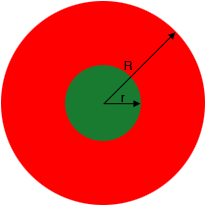SEARCH HOMEMath Central Quandaries & QueriesQuestion from Manasi, a student: The area between 2 concentric circles is 6 times the smaller circle. Radius of small circle is 7 cm. Find the difference in the circumference of the bigger circle and the smaller circle?Hi Manasi,

Suppose the radius of the smaller circle is $r$ and the radius of the larger circle is $R.$What is the area of the larger circle? What is the area of the smaller circle? The difference between these two areas is the area of the region between the circles. This area is 6 times the area of the smaller circle. Write this as an equation.

Substitute $r = 7$ cm and solve for $R.$

PennyMath Central is supported by the University of Regina and the Imperial Oil Foundation.